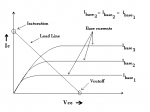# Common Emitter. Why and how toAs previously said, BJT's applications are typically as switcher or amplifier; switcher simply makes current flow or not - like light switch - and requires small reaction time, so that voltage goes from zero to max a value which gives a current matched on a curved line; and vice versa.

Amplifier mode needs Q-point lying on current curve when it becomes a ascending line, not curved.

In picture see three situations (from top to bottom): switcher, ambiguous, and amplifier.

Amplifying is possible only if Q-point stably stands on line, possibly in the middle.

Why?

Because this way we have the new voltage reference to make transistor work on signal variations: if we add to$V_{in}$ a AC voltage source input continuously moves up and down around$V_{in}$, and output voltage does same around Q-point.

By referring to graph, too big negative voltage leads transistor to switch off, while too big positive leads to over-current (not reported in graph) with a possible BJT burning.

Do you see now how Q-point's position is important?With a small AC signal (an AC signal is an Alternate one, which in a period of T seconds repeats its value, having average value zero in T range, like sine and cosine functions - see picture) transistor shows a particular behavior, so called differential; this is a mathematical concept here interpretable as transistor's changes based on little input variations.
That's what we're talking about, little AC signals - variations - added to$V_{in}$; how does it influence BJT?

Depending by kind of study there are two basic models: 1) π; 2) Giacoletto's. Let's see the first, and only it.
Again... differentiation is a special type of division but thought for infinitesimal changes. So:

• between B and E we have a little current$i_{b}$ based on little$v_{be}$ or$v_{\pi}$, and their ratio gives a resistance$r_{\pi}$;
• between C and E we have a little current$i_{c}$ controlled by$i_{b}$ or$v_{\pi}$ multiplied by correct coefficients (respectively$h_{fe}$ and$g_{m}$).Picture beside shows basic differential model, which reflects the two points above.
Pay attention to$h_{fe}$ (we don't actually need$g_{m}$); it's the current gain in differential mode: based on AC signal's frequency, it usually differs from β (gain in DC or continuous signal - the biasing).
They almost match when variation is so small to be considered negligible.
To clarify, sometimes you can read$h_{FE}$, with capital FE: this is same as β.
What does$h_{FE}$ or$h_{fe}$ stand for?!

1. h for hybrid; transistor can be represented as a 4-ports-net model (with two matching), where respective voltages and currents are multiplied by h-hybrid parameters, which have resistance, conductance or no-one dimensional value;
2. F and f -> forward (F for DC and f for AC);
3. E and e -> emitter (same).

Too much to say about equations characterization, but this would become too much mathematical or technical.
Anyway i hope this very quick introduction could stimulate your curiosity, knowing that if you now get on hands a BJT's datasheet, like 2n2222, you're able to distinguish a NPN from a PNP, and you know what bias and differential gains are.

Thanks!
As always see you on next article!